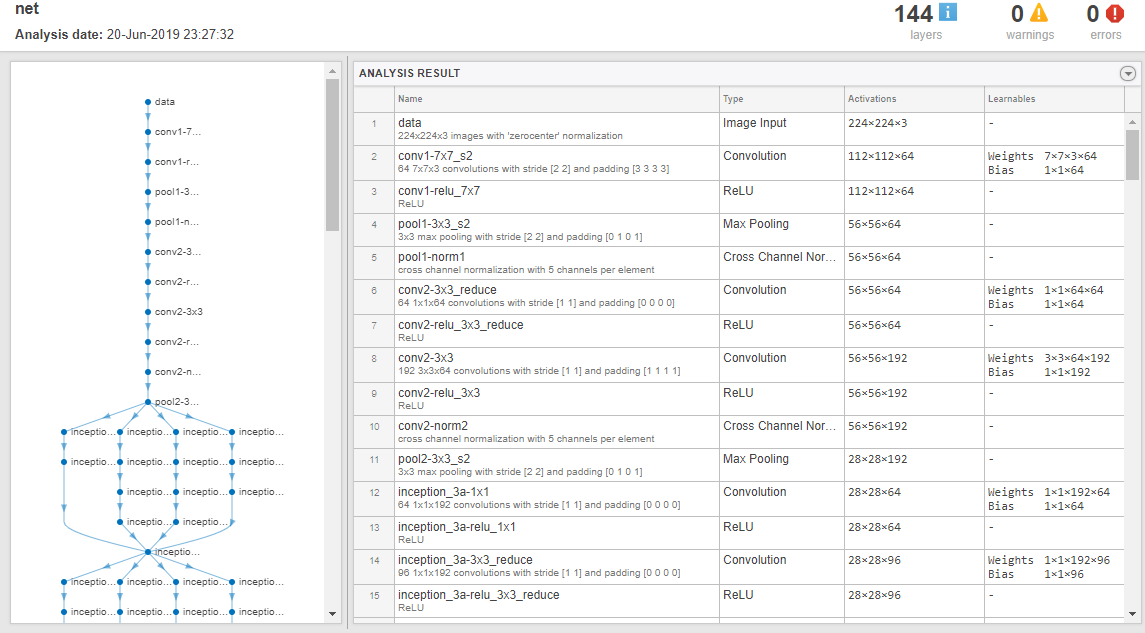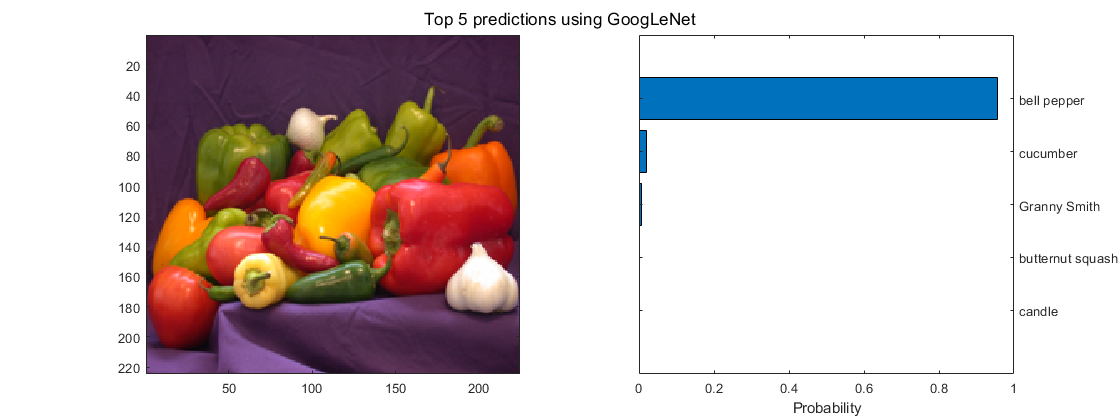## Code Generation for Deep Learning Networks by Using cuDNN

With GPU Coder™, you can generate optimized code for prediction of a variety of trained deep learning networks from Deep Learning Toolbox™. The generated code implements the deep convolutional neural network (CNN) by using the architecture, the layers, and parameters that you specify in the input `SeriesNetwork` (Deep Learning Toolbox) or `DAGNetwork` (Deep Learning Toolbox) object. The code generator takes advantage of NVIDIA® CUDA® deep neural network library (cuDNN) for NVIDIA GPUs. cuDNN is a GPU-accelerated library of primitives for deep neural networks. The generated code can be integrated into your project as source code, static or dynamic libraries, or executables that you can deploy to a variety of NVIDIA GPU platforms.

Generate code for convolutional networks by using one of the methods:

• The standard `codegen` function that generates CUDA code from a MATLAB® entry-point function.

• The GPU Coder app that generates CUDA code from a MATLAB entry-point function.

Note

In previous releases you could target the cuDNN library by using the `cnncodegen` function. From R2020b onwards, it is recommended to use the `codegen` command instead of the `cnncodegen` function because in a future release, the `cnncodegen` function will generate C++ code and build a static library for only the ARM® Mali GPU processor.

### Generate Code and Classify Images by Using GoogLeNet

In this example, you use GPU Coder to generate CUDA code for the pretrained `googlenet` (Deep Learning Toolbox) deep convolutional neural network and classify an image. GoogLeNet has been trained on over a million images and can classify images into 1000 object categories (such as keyboard, coffee mug, pencil, and animals). The network has learned rich feature representations for a wide range of images. The network takes an image as input, and then outputs a label for the object in the image together with the probabilities for each of the object categories. This example show you how to generate code for the pretrained network by using the `codegen` command and the GPU Coder app.

### Requirements

#### Required

This example generates CUDA MEX that has the following additional requirements.

1. Deep Learning Toolbox.

2. Deep Learning Toolbox Model for GoogLeNet Network support package.

3. GPU Coder Interface for Deep Learning Libraries support package.

4. CUDA enabled NVIDIA GPU and a compatible driver. For 8-bit integer precision, the CUDA GPU must have a compute capability of 6.1, 6.3 or higher.

#### Optional

For non-MEX builds such as static, dynamic libraries, or executables, this example has the following additional requirements.

1. CUDA toolkit and cuDNN libraries. For information on the supported versions of the compilers and libraries, see Installing Prerequisite Products.

2. Environment variables for the compilers and libraries. For more information, see Environment Variables.

1. Load the pretrained GoogLeNet network. You can choose to load a different pretrained network for image classification. If you do not have the required support packages installed, the software provides a download link.

```net = googlenet; ```

2. The object `net` contains the `DAGNetwork` object. Use the `analyzeNetwork` (Deep Learning Toolbox) function to display an interactive visualization of the network architecture, to detect errors and issues in the network, and to display detailed information about the network layers. The layer information includes the sizes of layer activations and learnable parameters, the total number of learnable parameters, and the sizes of state parameters of recurrent layers.

```analyzeNetwork(net); ```3. The image that you want to classify must have the same size as the input size of the network. For GoogLeNet, the size of the `imageInputLayer` (Deep Learning Toolbox) is 224-by-224-by-3. The `Classes` property of the output `classificationLayer` (Deep Learning Toolbox) contains the names of the classes learned by the network. View 10 random class names out of the total of 1000.

```classNames = net.Layers(end).Classes; numClasses = numel(classNames); disp(classNames(randperm(numClasses,10))) ```
``` 'speedboat' 'window screen' 'isopod' 'wooden spoon' 'lipstick' 'drake' 'hyena' 'dumbbell' 'strawberry' 'custard apple' ```

For more information, see List of Deep Learning Layers (Deep Learning Toolbox).

### Create an Entry-Point Function

1. Write an entry-point function in MATLAB that:

1. Uses the `coder.loadDeepLearningNetwork` function to load a deep learning model and to construct and set up a CNN class. For more information, see Load Pretrained Networks for Code Generation.

2. Calls `predict` (Deep Learning Toolbox) to predict the responses.

2. For example:

```function out = googlenet_predict(in) %#codegen persistent mynet; if isempty(mynet) mynet = coder.loadDeepLearningNetwork('googlenet'); end % pass in input out = predict(mynet,in); ```

A persistent object `mynet` loads the `DAGNetwork` object. At the first call to the entry-point function, the persistent object is constructed and set up. On subsequent calls to the function, the same object is reused to call `predict` on inputs, avoiding reconstructing and reloading the network object.

Note

Code generation requires the network to be loaded into a persistent object.

3. You can also use the `activations` (Deep Learning Toolbox) method to network activations for a specific layer. For example, the following line of code returns the network activations for the layer specified in `layerIdx`.

```out = activations(mynet,in,layerIdx,'OutputAs','Channels'); ```

4. You can also use the `classify` (Deep Learning Toolbox) method to predict class labels for the image data in `in` using the trained network, `mynet`.

```[out,scores] = classify(mynet,in); ```

For LSTM networks, you can also use the `predictAndUpdateState` (Deep Learning Toolbox) and `resetState` (Deep Learning Toolbox) methods. For usage notes and limitations of these method, see the corresponding entry in the Supported Functions table.

### Code Generation by Using `codegen`

1. To configure build settings such as output file name, location, and type, you create coder configuration objects. To create the objects, use the `coder.gpuConfig` function. For example, when generating CUDA MEX using the `codegen` command, use ```cfg = coder.gpuConfig('mex');```

Other available options are:

1. `cfg = coder.gpuConfig('lib');`, to create a code generation configuration object for use with `codegen` when generating a CUDA C/C++ static library.

2. `cfg = coder.gpuConfig('dll');`, to create a code generation configuration object for use with `codegen` when generating a CUDA C/C++ dynamic library.

3. `cfg = coder.gpuConfig('exe');`, to create a code generation configuration object for use with `codegen` when generating a CUDA C/C++ executable.

2. To specify code generation parameters for cuDNN, set the `DeepLearningConfig` property to a `coder.CuDNNConfig` object that you create by using `coder.DeepLearningConfig`.

```cfg = coder.gpuConfig('mex'); cfg.TargetLang = 'C++'; cfg.DeepLearningConfig = coder.DeepLearningConfig('cudnn'); cfg.DeepLearningConfig.AutoTuning = true; cfg.DeepLearningConfig.DataType = 'fp32'; ```

Specify the precision of the inference computations in supported layers by using the `DataType` property. When performing inference in 32-bit floats, use `'fp32'`. For 8-bit integer, use `'int8'`. Default value is `'fp32'`. `INT8` precision requires a CUDA GPU with minimum compute capability of 6.1. Use the `ComputeCapability` property of the `GpuConfig` object to set the appropriate compute capability value.

Note

Code generation for `INT8` data type does not support multiple deep learning networks in the entry-point function.

When performing inference in `INT8` precision using cuDNN version 8.1.0, issues in the NVIDIA library may cause significant degradation in performance.

3. Run the `codegen` command. The `codegen` command generates CUDA code from the `googlenet_predict.m` MATLAB entry-point function.

```codegen -config cfg googlenet_predict -args {ones(224,224,3)} -report ```

1. The `-report` option instructs `codegen` to generate a code generation report that you can use to debug your MATLAB code.

2. The `-args` option instructs `codegen` to compile the file `googlenet_predict.m` by using the class, size, and complexity specified for the input in. The value `(224,224,3)` corresponds to input layer size of the GoogLeNet network.

3. The `-config` option instructs `codegen` to use the specified configuration object for code generation.

Note

You can specify half-precision inputs for code generation. However, the code generator type casts the inputs to single-precision. The Deep Learning Toolbox uses single-precision, floating-point arithmetic for all computations in MATLAB.

The code generator uses column-major layout by default. To use row-major layout pass the `-rowmajor` option to the `codegen` command. Alternatively, configure your code for row-major layout by modifying the `cfg.RowMajor` parameter in the code generation configuration object.

4. When code generation is successful, you can view the resulting code generation report by clicking View Report in the MATLAB Command Window. The report is displayed in the Report Viewer window. If the code generator detects errors or warnings during code generation, the report describes the issues and provides links to the problematic MATLAB code. See Code Generation Reports.

```Code generation successful: View report ```

#### Generated Code

The DAG network is generated as a C++ class containing an array of 78 layer classes. The code generator reduces the number of layers by using layer fusion optimization of convolutional and ReLU layers. A snippet of the class declaration from `googlenet_predict_types.h` file is shown.

• The `setup()` method of the class sets up handles and allocates memory for each layer of the network object.

• The `predict()` method invokes prediction for each of the 78 layers in the network.

• The `DeepLearningNetwork.cu` file contains the definitions of the object functions for the `b_googlenet_0` class.

Binary files are exported for layers with parameters such as fully connected and convolution layers in the network. For instance, files `cnn_googlenet_conv*_w` and `cnn_googlenet_conv*_b` correspond to weights and bias parameters for the `FusedConvReLU` layers in the network. The code generator places these binary files in the `codegen` folder.

Note

On Windows® systems, some antivirus software such as Bit Defender can incorrectly identify some weight files as infected and delete them. These cases are false positives and the files can be marked as safe in your antivirus program.

In the generated code file `googlenet_predict.cu`, the entry-point function `googlenet_predict()` constructs a static object of b_googlenet_0 class type and invokes setup and predict on this network object.

### Generate Code by Using the App

To specify the entry-point function and specifying input types, complete the procedure in the app. See Code Generation by Using the GPU Coder App.

In the Generate Code step:

1. Set the `Build type` to `MEX`.

2. Click . In the Deep Learning pane, set Target library to cuDNN.3. Close the settings window. To generate CUDA code, click .

### Generated Makefile

For `'lib'`, `'dll'`, and `'exe'` targets, the code generator creates the `*_rtw.mk` make file in the `codegen` folder. In this make file, the location of the generated code is specified by using the `START_DIR` variable found in the `MACROS` section. By default, this variable points to the path of the current working folder where the code is generated. If you plan to move the generated files and use the makefile to build, replace the generated value of `START_DIR` with the appropriate path location.

### Run the Generated MEX

1. The image that you want to classify must have the same size as the input size of the network. Read the image that you want to classify and resize it to the input size of the network. This resizing slightly changes the aspect ratio of the image.

```im = imread("peppers.png"); inputLayerSize = net.Layers(1).InputSize; im = imresize(im,inputLayerSize(1:2)); ```
2. Call GoogLeNet predict on the input image.

```predict_scores = googlenet_predict_mex(im); ```
3. Display the top five predicted labels and their associated probabilities as a histogram. Because the network classifies images into so many object categories, and many categories are similar, it is common to consider the top-five accuracy when evaluating networks. The network classifies the image as a bell pepper with a high probability.

```[scores,indx] = sort(predict_scores, 'descend'); classNamesTop = classNames(indx(1:5)); h = figure; h.Position(3) = 2*h.Position(3); ax1 = subplot(1,2,1); ax2 = subplot(1,2,2); image(ax1,im); barh(ax2,scores(5:-1:1)) xlabel(ax2,'Probability') yticklabels(ax2,classNamesTop(5:-1:1)) ax2.YAxisLocation = 'right'; sgtitle('Top 5 predictions using GoogLeNet') ```